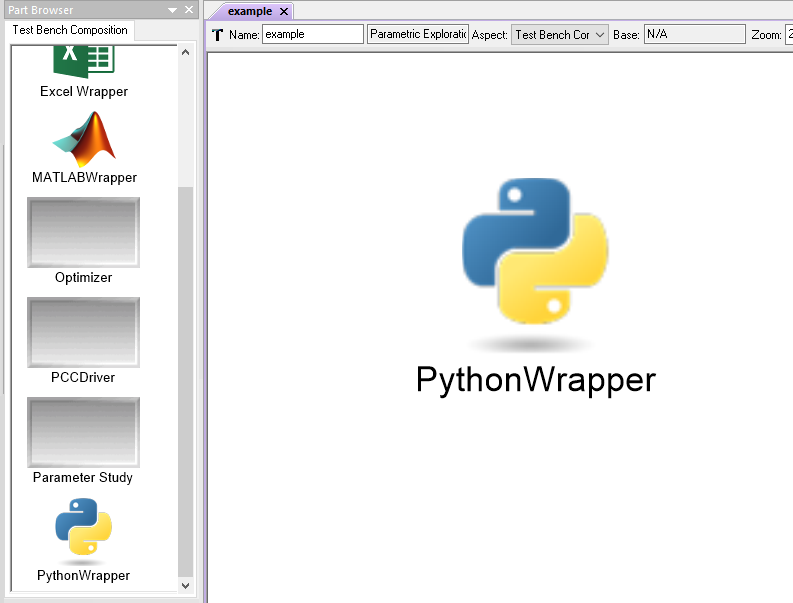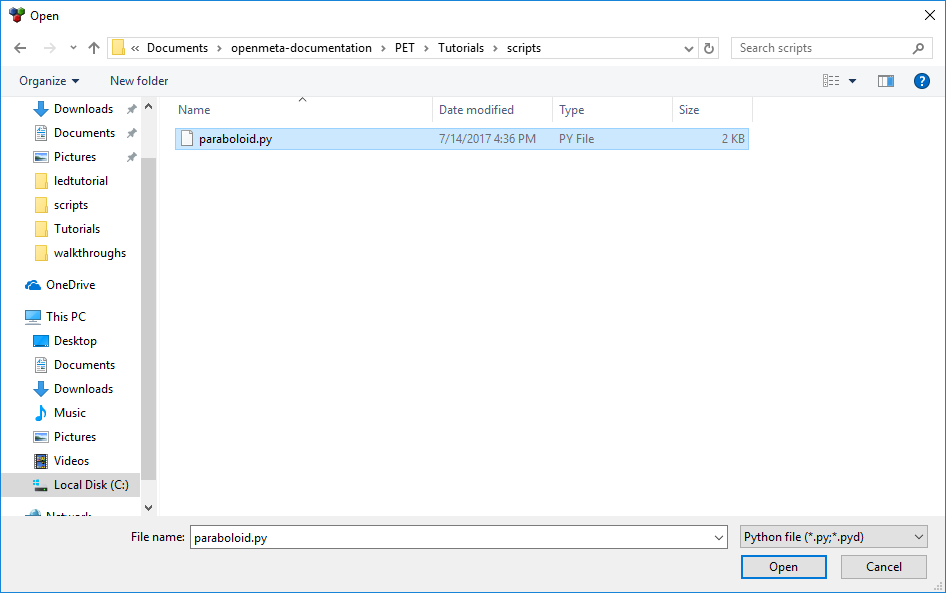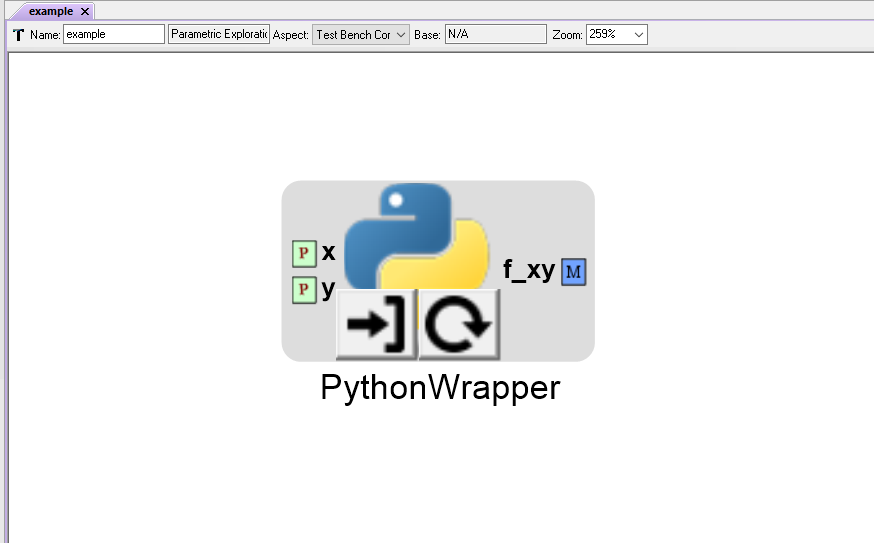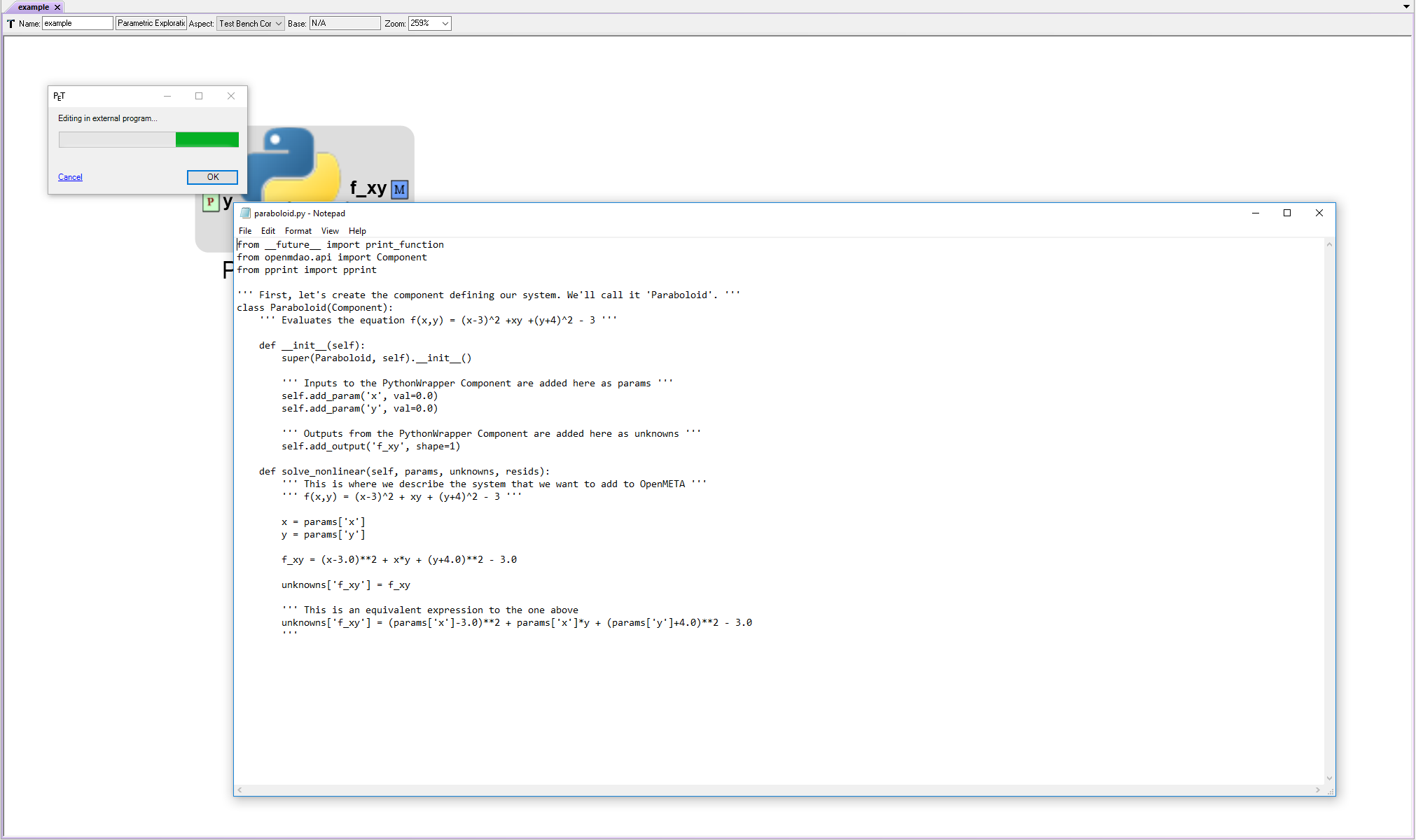# Python Wrappers¶

These serve as the most generic integration point. Practically any Python model or tool can be added to a PET using Python Wrappers.

## Adding Python Wrappers to a PET¶

To add a Python Wrapper to a PET, simply drag the Python Wrapper icon from the Part Browser and onto the PET canvas.A Python Wrapper in a PET

A Python Wrapper can be loaded with specially-formatted Python scripts.

Below is a template Python Wrapper OpenMDAO Component script:

Paraboloid.py
```from __future__ import print_function
from openmdao.api import Component
from pprint import pprint

''' First, let's create the component defining our system. We'll call it 'Paraboloid'. '''
class Paraboloid(Component):
''' Evaluates the equation f(x,y) = (x-3)^2 +xy +(y+4)^2 - 3 '''

def __init__(self):
super(Paraboloid, self).__init__()

''' Inputs to the Python Wrapper Component are added here as params '''

''' Outputs from the Python Wrapper Component are added here as unknowns '''

def solve_nonlinear(self, params, unknowns, resids):
''' This is where we describe the system that we want to add to OpenMETA '''
''' f(x,y) = (x-3)^2 + xy + (y+4)^2 - 3 '''

x = params['x']
y = params['y']

f_xy = (x-3.0)**2 + x*y + (y+4.0)**2 - 3.0

unknowns['f_xy'] = f_xy

''' This is an equivalent expression to the one above
unknowns['f_xy'] = (params['x']-3.0)**2 + params['x']*y + (params['y']+4.0)**2 - 3.0
'''
```

Note

For more information on OpenMDAO Component scripts and how to write them, reference the OpenMDAO documentation: http://openmdao.readthedocs.io/en/1.7.3/usr-guide/basics.html#component

To load a Python Wrapper, double-click on the Python Wrapper and use the file explorer to select the Python script to be added to the PET.A Python Wrapper loaded with a Python script

Note

You have to manually reload Python Wrappers whenever you change the exposed Params and Unknowns within the Python script.

Reloading also allows you to quickly switch between different versions of a Python script in your PET.

To load a Python Wrapper with a different Python script (or reload the same script), left-click on thebutton and select the desired Python file.To edit a Python Wrapper script from within OpenMETA, left-click on theicon